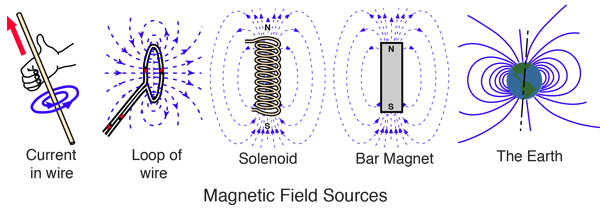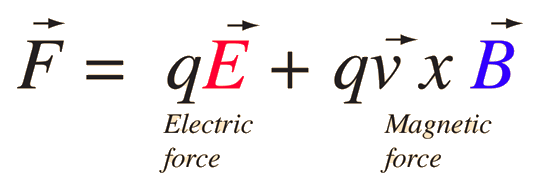# Magnetic Field

Magnetic fields are produced by electric currents, which can be macroscopic currents in wires, or microscopic currents associated with electrons in atomic orbits. The magnetic field B is defined in terms of force on moving charge in the Lorentz force law. The interaction of magnetic field with charge leads to many practical applications. Magnetic field sources are essentially dipolar in nature, having a north and south magnetic pole. The SI unit for magnetic field is the Tesla, which can be seen from the magnetic part of the Lorentz force law Fmagnetic = qvB to be composed of (Newton x second)/(Coulomb x meter). A smaller magnetic field unit is the Gauss (1 Tesla = 10,000 Gauss).Notes on units
Index

Electromagnetic force

Magnetic field concepts

 HyperPhysics***** Electricity and Magnetism R Nave
Go Back

# Lorentz Force Law

Both the electric field and magnetic field can be defined from the Lorentz force law:The electric force is straigtforward, being in the direction of the electric field if the charge q is positive, but the direction of the magnetic part of the force is given by the right hand rule.Index

Electromagnetic force

Magnetic force

 HyperPhysics***** Electricity and Magnetism R Nave
Go Back

# Magnetic Field Units

The standard SI unit for magnetic field is the Tesla, which can be seen from the magnetic part of the Lorentz force law Fmagnetic = qvB to be composed of (Newton x second)/(Coulomb x meter). A smaller magnetic field unit is the Gauss (1 Tesla = 10,000 Gauss).The magnetic quantity B which is being called "magnetic field" here is sometimes called "magnetic flux density". An older unit name for the Tesla is Webers per meter squared, with the Weber being the unit of magnetic flux.

 Magnetic field strength H
Index

Electromagnetic force

Magnetic field concepts

 HyperPhysics***** Electricity and Magnetism R Nave
Go Back